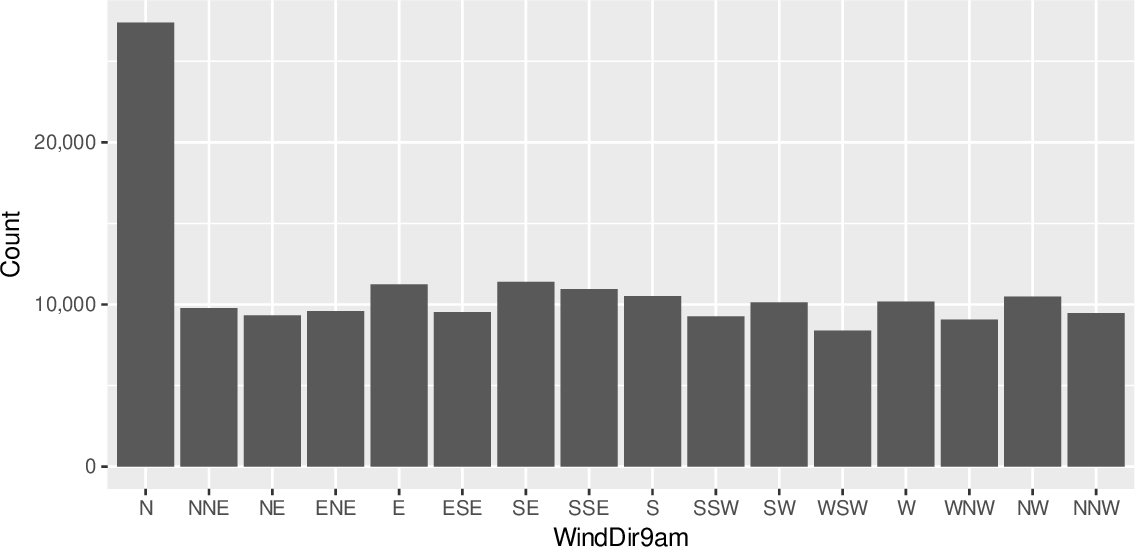Data Science Desktop Survival Guide by Graham WilliamsDesktop Survival Project Home Preface Data Science Introducing R R Constructs R Tasks R Strings R Read, Write, and Create Data Template Data Exploration Data Wrangling Data Visualisation Statistics ML Template ML Scenarios ML Activities ML Applications ML Algorithms Cluster Analysis Decision Trees Computer Vision Graph Data Privacy Literate Data Science Coding with Style Resources Bibliography Index

## Bar Chart Supplied Values

20200427ds %>%   count(wind_dir_9am) %>%   ggplot(aes(x=wind_dir_9am, y=n)) +   geom_col() +   scale_y_continuous(labels=comma) +   labs(x=vnames["wind_dir_9am"], y="Count") If the values of the bars are supplied in the source dataset (the y-axis) ggplot2::geom_col() is used. In this example we manually count the frequency of each wind direction in the dataset prior to invoking ggplot2::ggplot(). This modified dataset is then passed on to ggplot2::ggplot() to display. Of course, it would be better in this specific example to let ggplot2::geom_bar() do the work itself as in Section 10.8.